# 9. The cl-aa algorithm

Explanation of the cl-aa algorithm (the same algorithm used in the AntiGrain project.)

Consider a rasterizer whose coordinates accuracy is 1/4 of the pixel size. The following picture show a random triangle whose coordinates are not aligned to the grid (like when using the line-f function with random floating point coordinates):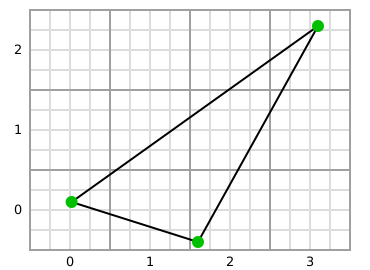The algorithm first aligns each of these points to the nearest grid interesection, as shown in this picture: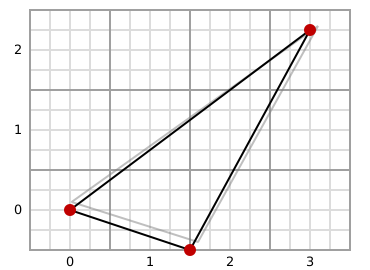Then it split each lines in the X axis at each grid boundary: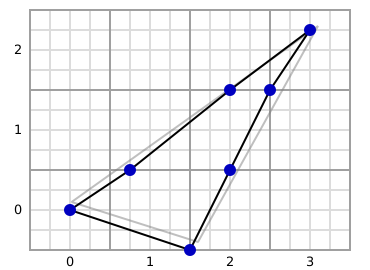Then the same operation is done in the Y axis: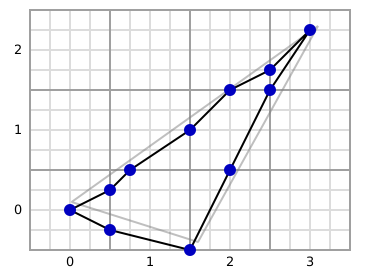At this stage, we can see that there is a loss of precision. However, cl-aa use 1/256 subpixel accuracy by default, which is largely enough usually.

After this step, cl-aa look at each line segment (each line between 2 dots) to compute the (signed) area from the segment to the left border (storing in fact twice the area for optimization purpose) and to compute "cover" which is simply the height of the segment. For a same line of pixels, cover must cancel when accumulating the value from the leftmost cell to the rightmost one. This happen when the polygon is closed, and is expected for the algorithm to work properly.

Scanning these segments for each cell gives the following: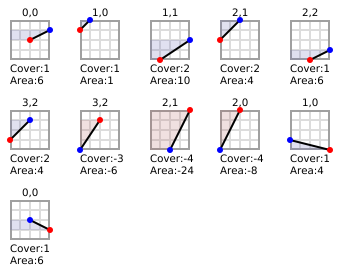(The algorithm really only keep area and cover values per cell, and discard segment coordinates.)

The red dot is the start of the segment (assuming the triangle was described in clockwise direction, starting from the point at the left.) The area is colored in blue when it is positive, and colored in red when it is negative. As said above, the area is in fact twice the real value.

Once sorted by Y then by X, we obtain: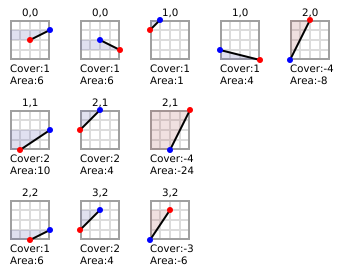As you can see, the sum of cover value cancels at the end of each line.

Then cells-sweep process each line of cells accumulating area for cells with the same coordinates, and accumulating cover along the whole line, and computing the effective area for each pixels with the following formula (where width is the accuracy of subpixel coordinate, which is 4 in our examples). The term area and cover are borrowed from the AntiGrain algorithm.

```                                 area
effective-area = width x cover - ----
2
```

The effective area is then scaled, usually to the range (0-256), and passed to the callback function provided to cells-sweep.

As an example, we consider the line 2 (the 3 cells in the bottom of the last picture.) The effective area for the cell at (2,2) is 4 x 1 - 6 / 2 = 1 (which can be verified visually.) Then we have 2 cells at (3,2). We sums their values, which gives us -2 for area and -1 for cover. Accumulating cover with the previous cells cancels (as expected since it is the last pixel on this line), which gives an effective area at (3,2) of 4 x 0 - (-2) / 2 = 1 (which is perhaps less obvious to check visually.)

If two consecutive cells have a hole (i.e. x coordinates difference is superior to 1), each intermediate cells have implicitly an area of 0 and a cover of 0, and thus an accumulated cover which is constant along this span of implicit cells.

FIXME: Update the example to have a hole between 2 cells.

Generated by CL-Crock on 2010-09-25T15:20:59Z